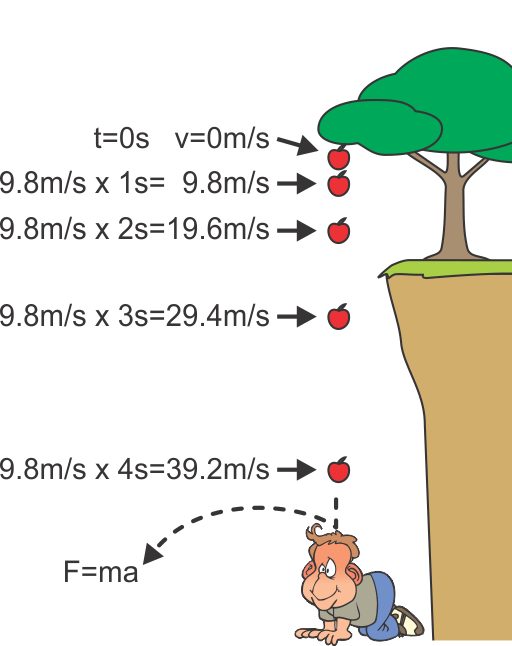Crystal

HS-PS2-1

# Acceleration Due To Gravity Study Guide

All the bodies on the earth face a constant force and acceleration due to gravity.

# INTRODUCTION

What do you expect when you drop a feather and a brick at the same time? The answer is that they will all fall at the exact same time; though some objects, like feathers, seem to fall slower because of air resistance. In order to see the true nature of gravity affecting the feathers, you need to remove all the air in the room. The reason behind this is that in a vacuum, both will face equal acceleration due to gravity!Source

## ACCELERATION AND GRAVITY

• The meaning of acceleration is basically any process in which the velocity (which is a measure of speed and the direction of motion of a body) varies with time.

• Mathematically represented as dv/dt (change in velocity/time period), it is a vector quantity and can be positive, negative, or zero depending upon the velocity and its direction.

• The meaning of gravity, on the other hand, is the force that pulls an object towards the center of the earth.

• F= mg is the gravitational force acting on a body,

where f is the force acting on a body,

m is its mass,

and g is the acceleration due to gravity.

## ACCELERATION DUE TO GRAVITY

• The further an object descends forward towards the bottom, more gravity causes it to drop at a quicker and faster rate.
• In practice, the velocity of an object increases by 9.8 m/s2 ( the value of the acceleration due to the gravity on earth ).
• Therefore an object's velocity is 9.8 m/s 1 second after it begins to fall.
• Its velocity will further keep on increasing with time because of the acceleration due to gravity.
• The SI unit of acceleration due to gravity is the same as that of the acceleration, m/s2.Source

The universal law of gravitation states that f = GmM/(r+h)2

Where f denotes the force between two bodies

G (6.6710-11 Nm2/kg2) is the universal gravitational constant

m is the object's mass

M is the earth's mass

r is the earth's radius and

h = the distance between the body and the earth's surface.

As the height would be very less when compared with the radius of the earth

f = GmM/r2

On comparing both the equations, we get

g = GM/r2, the formula for the acceleration due to gravity.

This enables us to comprehend the following:

• Gravity accelerates all bodies at the same rate, regardless of their mass.
• Its value on Earth is determined by the mass of the planet, not really the mass of the item.

# Summary

• Acceleration is the process in which the velocity of a body varies with time.
• Gravity is the force that pulls an object towards the center of the earth.
• The value of the acceleration due to the gravity on earth is 9.8 m/s2.
• g = GM/r2 is the equation used to calculate acceleration due to gravity.

## FAQs

1. What is acceleration due to gravity independent of?

Acceleration due to gravity is independent of the mass of the body.

2. What is the unit of acceleration due to gravity?

The SI unit of the acceleration due to gravity is the same as that of the acceleration, m/s2.

3. How do you calculate acceleration due to gravity?

We can calculate acceleration due to gravity with the expression g = GM/r2.

4. What is the equation for acceleration due to gravity?

g = GM/r2 is the equation used for acceleration due to gravity

We hope you enjoyed studying this lesson and learned something cool about Acceleration Due To Gravity! Join our Discord community to get any questions you may have answered and to engage with other students just like you! We promise, it makes studying much more fun!😎

## REFERENCE

1. Acceleration Due to Gravity: https://www.ck12.org/physics/acceleration-due-to-gravity/lesson/Acceleration-Due-to-Gravity-MS-PS/?referrer=concept_details. Accessed 8th April 2022.
2. Acceleration Due to Gravity: https://www.vedantu.com/iit-jee/acceleration-due-to-gravity Accessed 8th April 2022